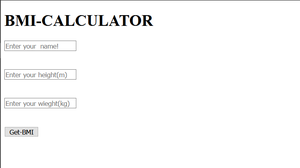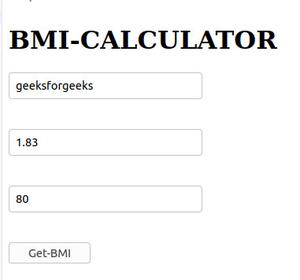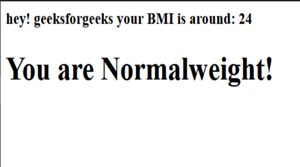# BMI Calculator using Express.js

• Difficulty Level : Medium
• Last Updated : 13 Oct, 2021

The Body Mass Index(BMI) is represented in terms of weight and height of an individual. It is the ratio of the weight of the body to the square of the height of the body in kilograms and meters respectively.

```BMI = (weight of body) / (height of body)2
Unit of weight: Kilogram(Kg);
Unit of height: Meter(m);
Unit of BMI is kg/m2```

Approach:

• First, we write HTML code for creating a form in which we will take name, height(m), weight(kg) as input from the user.
• Import require modules and store it into app variable
• sends html and post the data on the specific route using this

```app.post("/bmicalculator", function (req, res) {
heigh = parseFloat(req.body.Height);
weigh = parseFloat(req.body.Weight);
bmi = weigh / (heigh * heigh);```
• Check Condition for BMI using formula.

Step-1:

## HTML

 ```<``html` `lang``=``"en"``>``    ``<``head``>``        ````        ``<``title``>BMI-CALCULATOR``        ````        ``<``link` `rel``=``"stylesheet"` `href``=``"1.css"` `/>``    ````    ``<``body``>``        ``<``div` `id``=``"MAIN"``>``            ``<``h1` `id``=``"heading"``>BMI-CALCULATOR``        ``` `        ``<``form` `action``=``"/bmicalculator"` `method``=``"post"``>``            ``<``input` `type``=``"text"``                   ``name``=``"Name"``                   ``placeholder``=``"Enter your  name!"` `/>``            ``<``br` `/>``            ``<``input` `id``=``"Height"``                   ``name``=``"Height"``                   ``placeholder``=``"Enter your height(m)"` `/>``            ``<``br` `/>``            ``<``input` `id``=``"Weight"``                   ``name``=``"Weight"``                   ``placeholder``=``"Enter your weight(kg)"` `/>``            ``<``br` `/>``            ``<``button` `class``=``"btn"``                    ``type``=``"submit"``>Get-BMI``        ````    `````

Output:SIMPLE FORM

Let’s now write code for our calculations and functionalities which is the main part of our BMI-CALCULATOR.

Step-2

```Dependencies:
express:    npm install express
bodyparser:   npm install body-parser```

## Javascript

 `//importing modules``const express = require(``"express"``);``const bodyparser = require(``"body-parser"``);` `// stores the express module into the app variable!``const app = express();``app.use(bodyparser.urlencoded({ extended: ``true` `}));` `//sends index.html``app.get(``"/bmicalculator"``, ``function` `(req, res) {``    ``res.sendFile(__dirname + ``"/"` `+ ``"index.html"``);``});` `//this is used to post the data on the specific route``app.post(``"/bmicalculator"``, ``function` `(req, res) {``    ``heigh = parseFloat(req.body.Height);``    ``weigh = parseFloat(req.body.Weight);``    ``bmi = weigh / (heigh * heigh);` `    ``//number to string format``    ``bmi = bmi.toFixed();` `    ``req_name = req.body.Name;` `    ``// CONDITION FOR BMI``    ``if` `(bmi < 19) {``        ``res.send(``"

hey! "` `+ req_name +``                 ``" your BMI is around: "` `+ bmi +``                 ``"

You are Underweight!"``);``    ``} ``else` `if` `(19 <= bmi && bmi < 25) {``        ``res.send(``"

hey! "` `+ req_name +``                 ``" your BMI is around: "` `+ bmi +``                 ``"

You are Normalweight!"``);``    ``} ``else` `if` `(25 <= bmi && bmi < 30) {``        ``res.send(``"

hey! "` `+ req_name +``                 ``" your BMI is around: "` `+ bmi +``                 ``"

You are Overweight!"``);``    ``} ``else` `{``        ``res.send(``"

hey! "` `+ req_name +``                 ``" your BMI is around: "` `+ bmi +``                 ``"

You are Obese!"``);``    ``}``});` `//this is used to listen a specific port!``app.listen(7777, ``function` `() {``    ``console.log(``"port active at 7777"``);``});`

Output:Final Output:Output

My Personal Notes arrow_drop_up